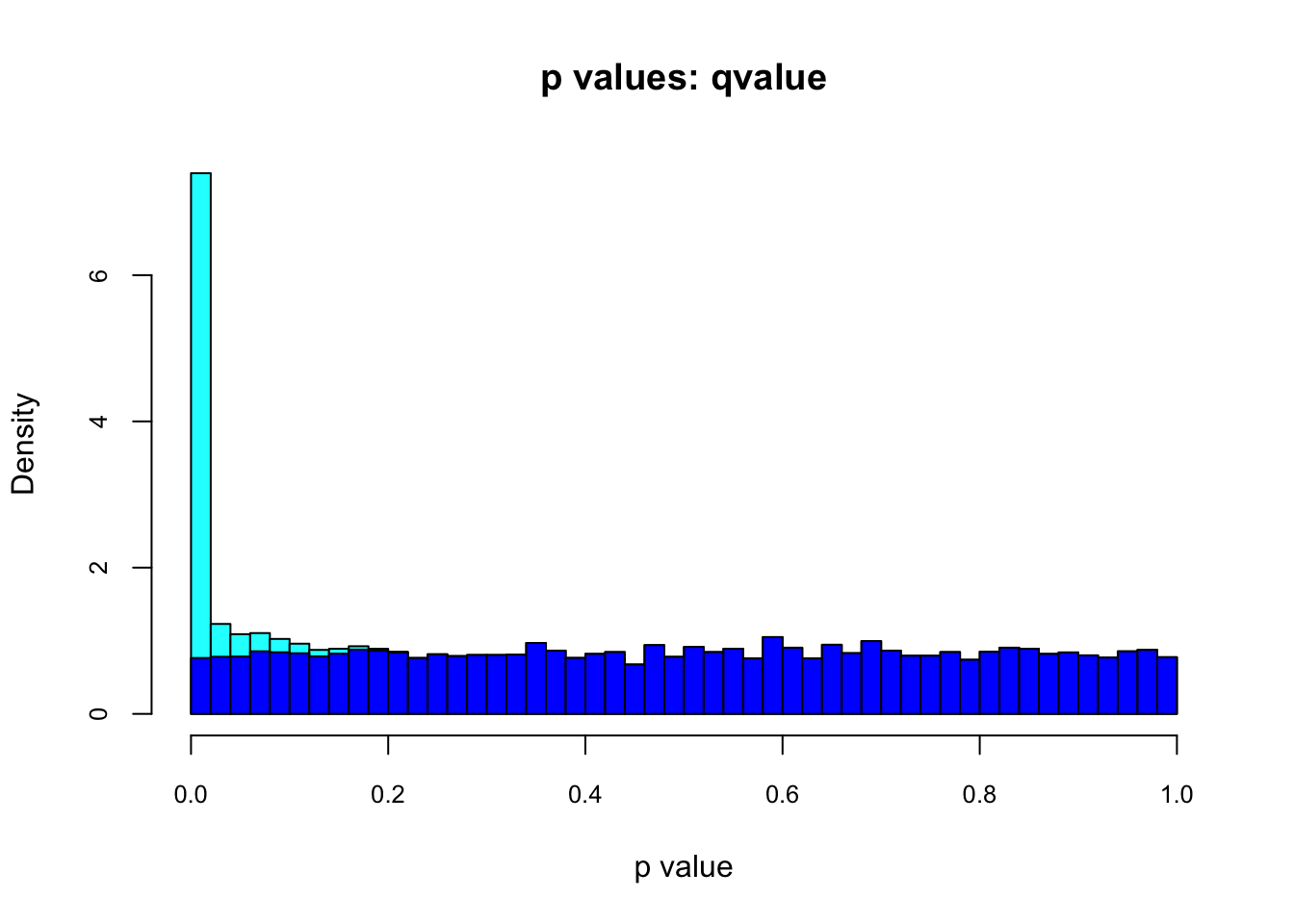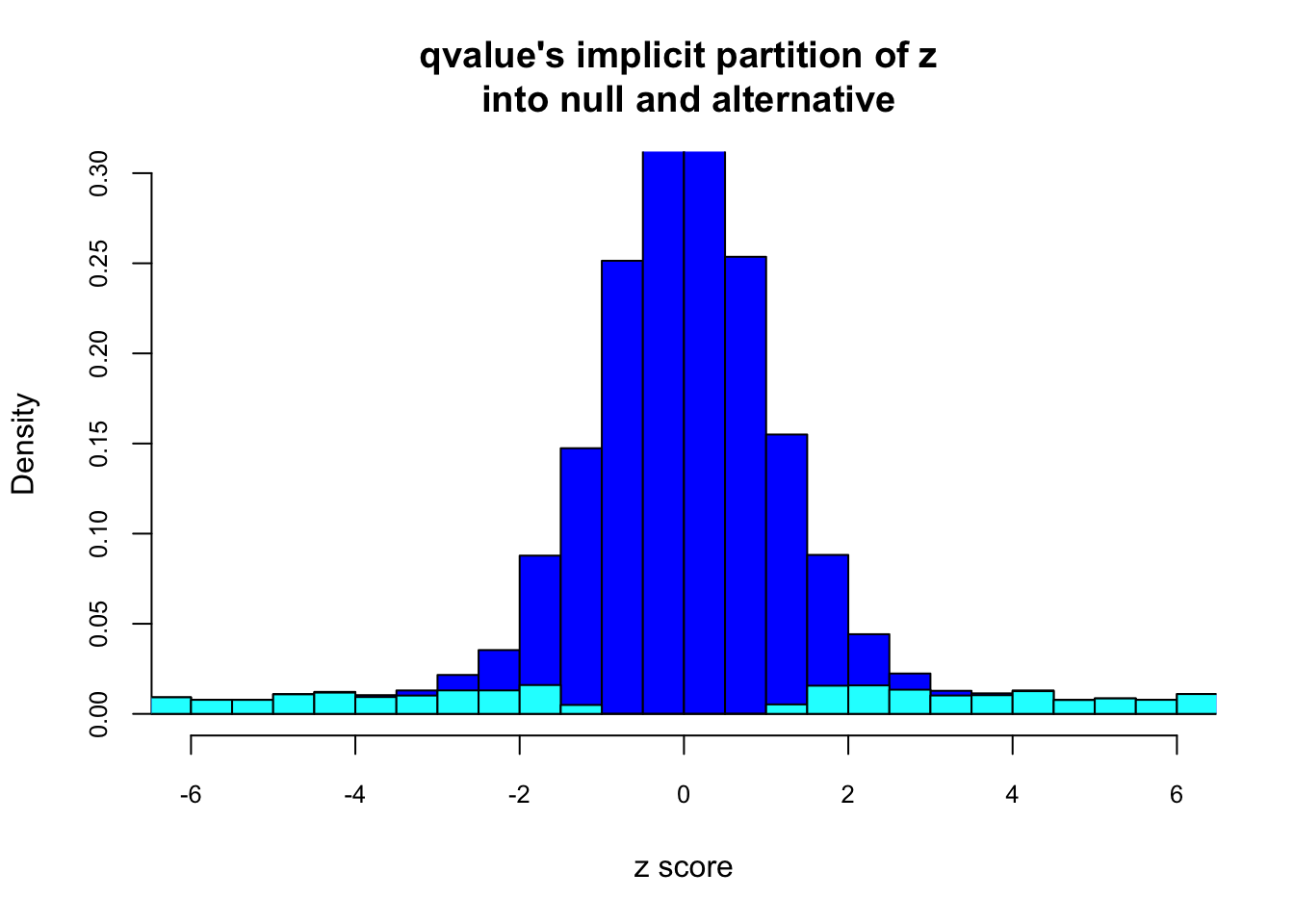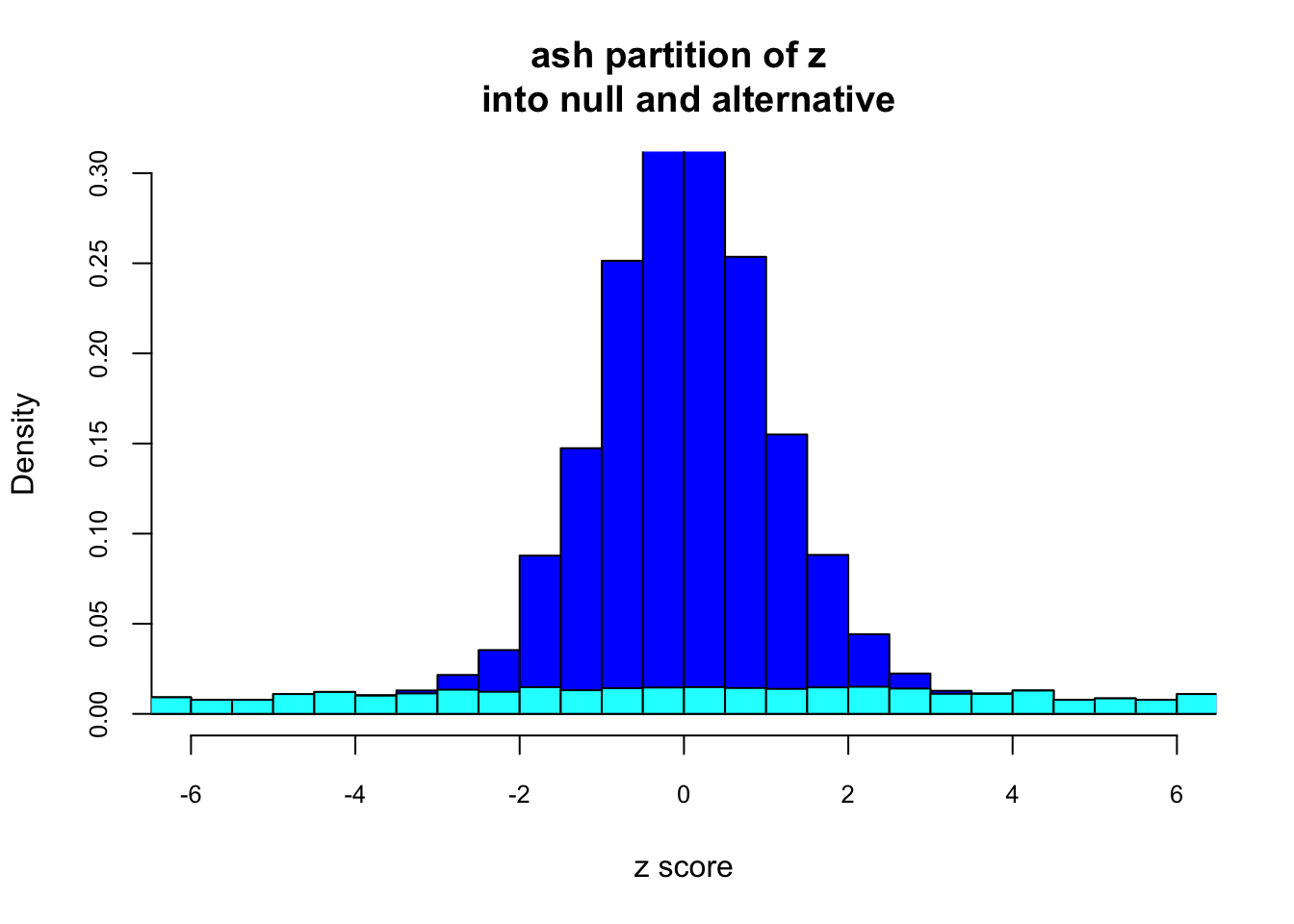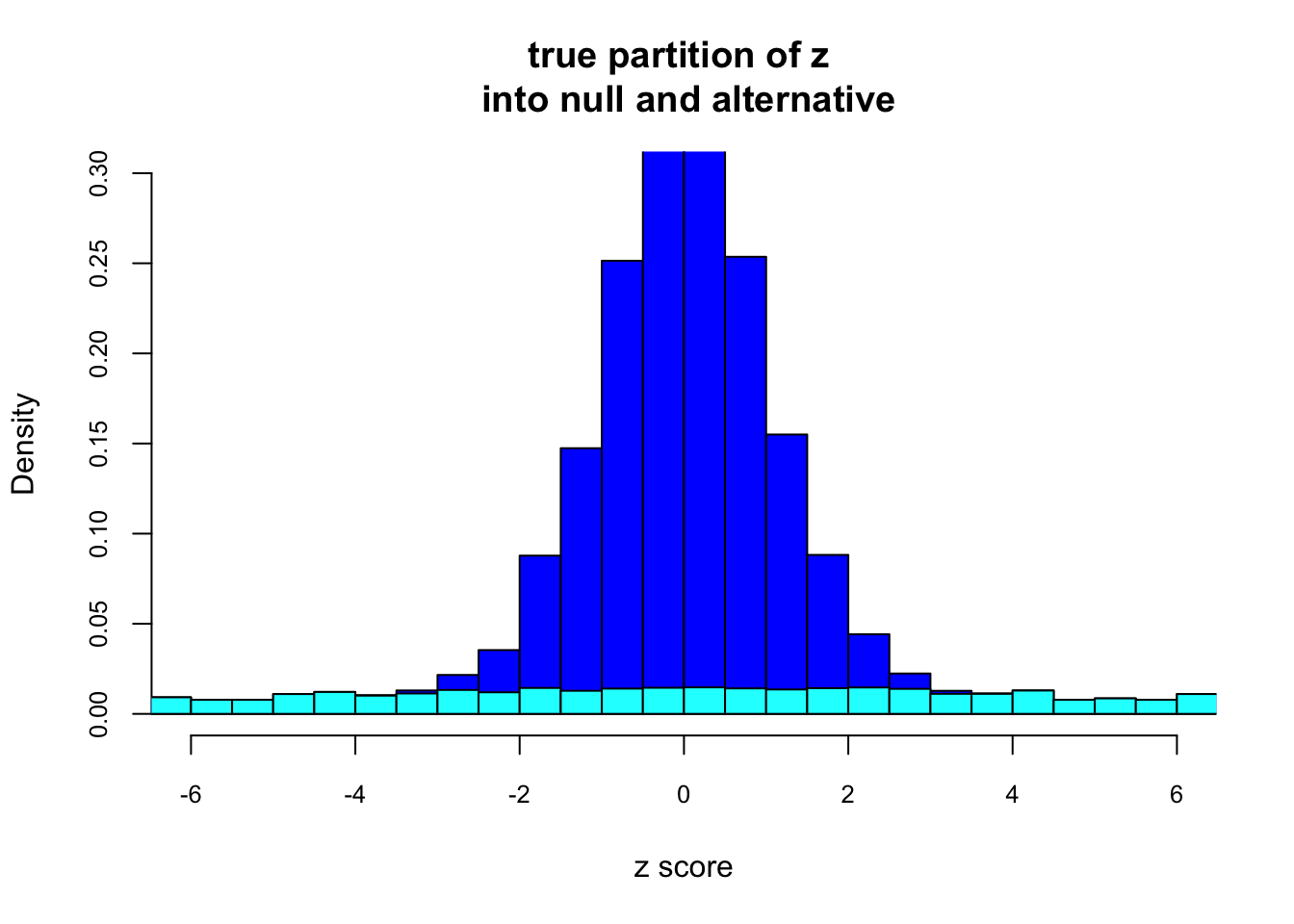Last updated: 2016-12-09

Code version: 263cb495f719be371368abbbfa59f066e8482fba

A referee reported a simulation of 10,000 hypotheses, a fraction 0.8 are null ($$\mu_i = 0$$), and a fraction 0.2 are alternative with effect $$\mu_i \sim N(0,1)$$. For each effect $$i$$ there are n=30 observations from $$x_{i,j} \sim N(\mu_i,1)$$ and a one-sample t test is used to compute a $$p$$ value. The referee correctly notes that these simulations i) obey the unimodal assumption (UA), and ii) have the property that “most” $$z$$ scores near 0 are null (Efron’s “Zero Assumption”; ZA). The motivation is to demonstrate that the UA and the ZA are not contradictory.

I agree with this, if we define the ZA as “most” $$z$$ scores near 0 are null. However, in practice the existing methods effectively make a stronger assumption (or at least, behave as if they do): that all $$z$$ scores near 0 are null. Consequently they end up creating a hole in the distribution of alternative $$z$$ scores at 0. Here we illustrate this by applying qvalue to this scenario.

First, we load the necessary libraries, and specify settings for displaying the plots in the rendered document.

## Simulation

First, we generate a small data set.

set.seed(100)
mu = c(rep(0,8000),rnorm(2000))
x = matrix(rnorm(30*10000),nrow=10000,ncol=30)+mu
ttest.pval = function(x){return(t.test(x)$p.value)} x.p = apply(x,1,ttest.pval) Here is how qvalue (arguably, implicitly) decomposes the distribution of p values into null and alternative source("../R/nullalthist.R") lfdr.qv = qvalue::qvalue(x.p)$lfdr
altnullhist(x.p,lfdr.qv,main="p values: qvalue",ncz=40,xlab="p value",cex.axis=0.8)And here is the corresponding plot in z score space. Note the “hole” in the distribution of $$z$$ scores at 0 under the alternative.

ttest.est = function(x){return(t.test(x)\$estimate)}
x.est = apply(x,1,ttest.est)
zscore = x.est/(1/sqrt(30))
nullalthist(zscore,lfdr.qv,main="qvalue's implicit partition of z \n into null and alternative",ncz=60,cex.axis=0.8,ylim=c(0,0.3),xlim=c(-6,6),xlab="z score")For comparison here is the decomposition, using ash, of $$z$$ scores into null and alternative components.

library(ashr)
lfdr.ash=get_lfdr(ash(x.est,1/sqrt(30)))
nullalthist(zscore,lfdr.ash,main="ash partition of z \n into null and alternative",ncz=60,cex.axis=0.8,ylim=c(0,0.3),xlim=c(-6,6),xlab="z score")For completeness here is (roughly) the true" decomposition of $$z$$ scores into null and alternative components.

trueg = ashr::normalmix(c(0.8,0.2),c(0,0),c(0,1))
lfdr.true=get_lfdr(ash(x.est,1/sqrt(30),g=trueg,fixg=TRUE))
nullalthist(zscore,lfdr.true,main="true partition of z \n into null and alternative",ncz=60,cex.axis=0.8,ylim=c(0,0.3),xlim=c(-6,6),xlab="z score")## Explanation

In estimating $$\pi_0$$ qvalue assumes that all p values near 1 are null. This is equivalent to assuming that all z scores near 0 are null. Thus, despite the fact that qvalue does not explicitly model the distribution of $$p$$ values or $$z$$ scores under the alternative, its way of estimating $$\pi_0$$ necessarily creates a hole in the $$z$$ score distribution at 0 under the alternative.

## Session information

sessionInfo()
R version 3.3.1 (2016-06-21)
Platform: x86_64-apple-darwin13.4.0 (64-bit)
Running under: OS X 10.12.1 (Sierra)

locale:
 en_US.UTF-8/en_US.UTF-8/en_US.UTF-8/C/en_US.UTF-8/en_US.UTF-8

attached base packages:
 stats     graphics  grDevices utils     datasets  methods   base

other attached packages:
 ashr_2.0.4 knitr_1.14

loaded via a namespace (and not attached):
 Rcpp_0.12.8       magrittr_1.5      REBayes_0.63
 MASS_7.3-45       splines_3.3.1     pscl_1.4.9
 munsell_0.4.3     doParallel_1.0.10 lattice_0.20-33
 SQUAREM_2016.8-2  colorspace_1.2-6  foreach_1.4.3
 stringr_1.0.0     plyr_1.8.4        tools_3.3.1
 parallel_3.3.1    grid_3.3.1        gtable_0.2.0
 iterators_1.0.8   htmltools_0.3.5   assertthat_0.1
 yaml_2.1.13       rprojroot_1.1     digest_0.6.10
 Matrix_1.2-6      reshape2_1.4.1    ggplot2_2.1.0
 formatR_1.4       codetools_0.2-14  qvalue_2.4.2
 evaluate_0.9      rmarkdown_1.2     stringi_1.1.1
 Rmosek_7.1.3      scales_0.4.0      backports_1.0.4
 truncnorm_1.0-7